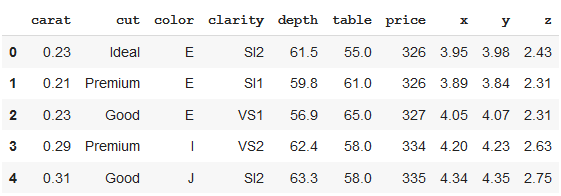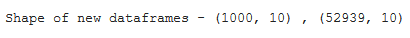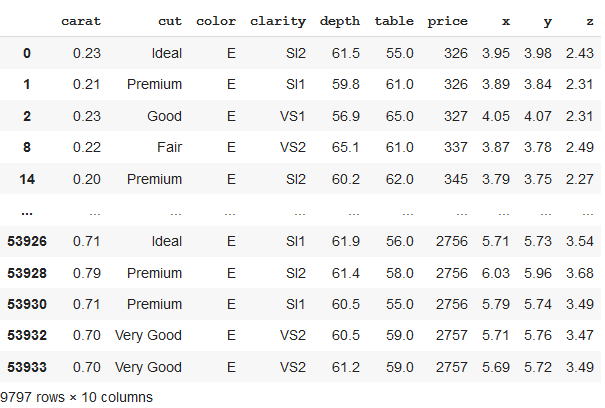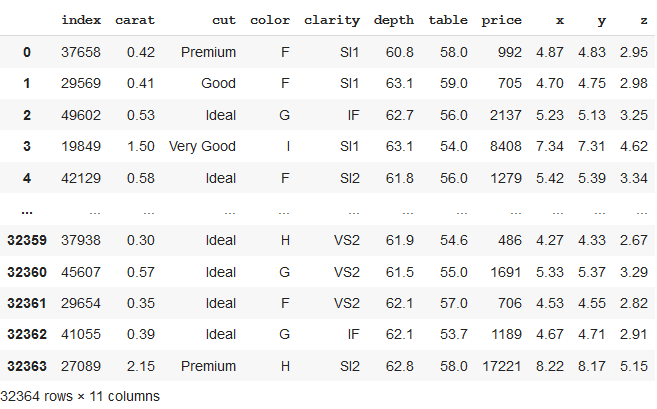GeeksforGeeks App
Open AppBrowser
Continue

# Split Pandas Dataframe by Rows

We can try different approaches for splitting Dataframe to get the desired results. Let’s take an example of a dataset of diamonds.

## Python3

 `# importing libraries``import` `seaborn as sns``import` `pandas as pd``import` `numpy as np` `# data needs not to be downloaded separately``df  ``=` `sns.load_dataset(``'diamonds'``)``df.head()`

Output:Method 1: Splitting Pandas Dataframe by row index
In the below code, the dataframe is divided into two parts, first 1000 rows, and remaining rows. We can see the shape of the newly formed dataframes as the output of the given code.

## Python3

 `# splitting dataframe by row index``df_1 ``=` `df.iloc[:``1000``,:]``df_2 ``=` `df.iloc[``1000``:,:]``print``(``"Shape of new dataframes - {} , {}"``.``format``(df_1.shape, df_2.shape))`

Output:Method 2: Splitting Pandas Dataframe by groups formed from unique column values
Here, we will first grouped the data by column value “color”. The newly formed dataframe consists of grouped data with color = “E”.

## Python3

 `# splitting dataframe by groups``# grouping by particular dataframe column``grouped ``=` `df.groupby(df.color)``df_new ``=` `grouped.get_group(``"E"``)``df_new`

Output:Method 3 : Splitting Pandas Dataframe in predetermined sized chunks
In the above code, we can see that we have formed a new dataset of a size of 0.6 i.e. 60% of total rows (or length of the dataset), which now consists of 32364 rows. These rows are selected randomly.

## Python3

 `# splitting dataframe in a particular size``df_split ``=` `df.sample(frac``=``0.6``,random_state``=``200``)``df_split.reset_index()`

Output:My Personal Notes arrow_drop_up